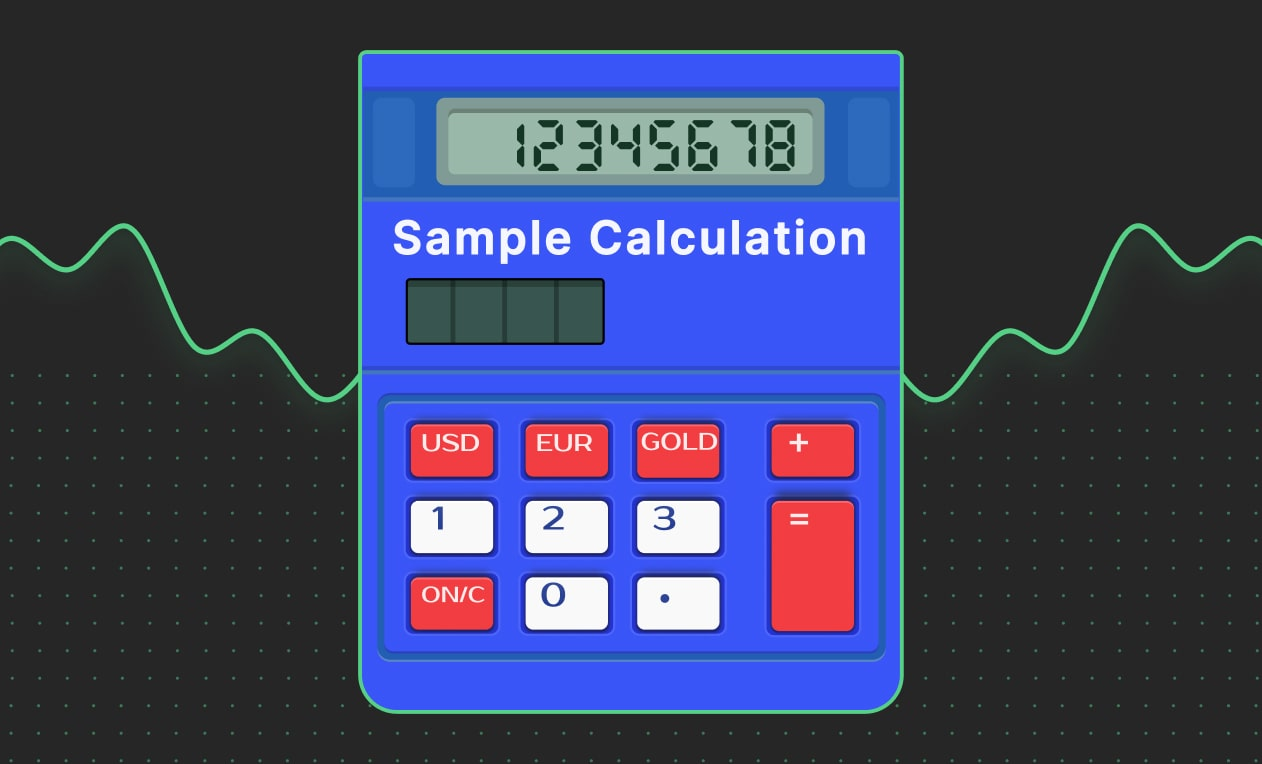# Dividends on margin

## Maximize Earnings with dividends on margin:

activate program, keep your opened positions overnight and earn weekly.

# Profit from open positions

Dividend rate 10% 20% 15%

## Dividend rate

Micro

10%

20%

Pro

15%

Annual dividend rate is calculated daily at 00:00 server time, and paid out weekly on your trading account. Annual dividends do not apply to Copy Trading accounts and Swap free accounts. Terms and conditions applied.

## Dividends on margin Sample Calculation

### Scenario

Consider a 2-lot sell trade on the EURUSD on a Premium account that remains open at 00:00 server time. The anticipated margin for this trade is 2135.14 USD*.

### Daily Dividends Calculation

Computed daily dividends at an annual rate of 20% (for Premium accounts) over 365 days would be

20%/ 365 * 2135.14 = 1.17 USD*Please note that the margin is an estimate, and the precise value can be determined by using the trader's calculator.

**Kindly be aware that the Swap value varies across different types of instruments. For additional information, please refer to theTrading Instruments tab.

## Dividends on margin FAQ

### When dividend rate is calculated?

The annual dividend rate is calculated daily at 00:00 server time.

### When accumulated dividends payments are made?

Dividends payments are made weekly on Mondays. The weekly dividends amount is calculated as the sum of daily dividends payments for the past week.

### What's the formula for dividends calculation?

Daily Dividends = Annual Dividends / 365 * Used Margin, where Used Margin is the total amount allocated to open positions in the trading account.

### For which accounts the dividend rate is calculated?

The dividend rate is calculated for real Micro, Premium, and Pro accounts that do not participate in copy trading and have swaps enabled.

### What are the dividend rates for different account types?

• Micro - 10% (annual)
• Pro - 15% (annual)

### Is the dividend rate applied if the client only trades with bonus funds (without real funds in the account)?

There is no dividend rate on trading with bonus funds only.

### Where accumulated dividends payments are deposited?

Dividends payments are directly deposited into clients' real trading accounts.

### Does the dividend rate apply to trades opened and closed within one day?

The annual dividend rate is calculated daily at 00:00 server time and only applies to the used margin at that time. If the margin was used during the day but orders were closed before 00:00, it will not be considered.

### Does the dividend rate apply to trades open for multiple days (e.g., 2 days)?

If a client has a trade opened at 00:00 on the first day and it remains open until 00:00 on the following day, the dividends will be calculated on the used margin twice: on the first and the second day.

### How to participate in the Program?

• The client needs to verify his phone number.
• Activate the program.
• Open a real swap account (Micro, Premium or Pro).

### What is the minimum payout amount?

The minimum payout amount to the account is 1 cent.

scroll up